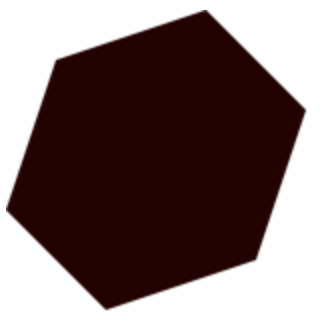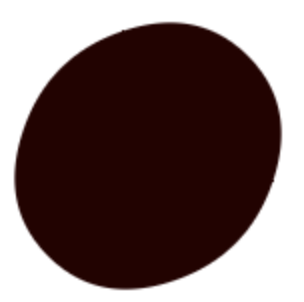[ frain ]## What's the problem?

I had a problem drawing polygons and smoothing the edges of it, a.k.a Curve Fitting

When I learned how to do this, I used PIXI JS but you can easily achieve the same by using the 2d context given from a canvas, which I will be doing in this post.

So we're going to work with a set of points. Those will be

``````const polygonPoints = [
[25, 25],
[100, 0],
[150, 50],
[125, 125],
[50, 150],
[0, 100]
];
``````

You can see these as the original points, which are quite rough.

So the next step is probably to render these points, right? Let's do that.

``````const canvas = document.querySelector('#glCanvas');
// Initialize GL Context
const context = canvas.getContext('2d');

context.fillStyle = 'rgb(40,0,0)';

polygonPoints.forEach(([x, y], i) => {
if(i === 0) context.moveTo(x, y);
context.lineTo(x, y);
})

context.fill();
``````

That should leave us with a result like this:#### Sure, it's drawing a polygon

• but it's quite rough.

So now let's spice things up by using the quadraticCurveTo

What it essentially does is, it draws a curve between given points based on the angle but also the distance between the two points. Sounds like something we want to do if we think the polygon is too rough.

But this also means we have to alter the points in some way, since we're not after a shape with straight lines anymore, the points will be moved. The gotcha in this is the quadraticCurveTo, it will help us with all of that BUT the first and the last point, since it does not know we're drawing a closed shape, it thinks we're drawing a curved line.

Try this example:

``````polygonPoints
.map((p) => ({ x: p, y: p }))
.forEach((point, i, points) => {
const nextPoint = points[(i + 1) % points.length];
if(i === 0){
context.moveTo(point.x, point.y);
}

point.x,
point.y,
(point.x + nextPoint.x) / 2,
(point.y + nextPoint.y) / 2,
);
});
``````

What's happening here? We're using the current iterations point as control points, and the next points are half the distance between the control and the next point, unless the index is 0 or the last in the array. As I mentioned quadraticCurveTo is mainly for doing this on a straight line and it will not affect the first or the last points.

So how do we solve this?

One way is to move the pen to the "correct" starting point. And that would be half the distance between the last and the first point, like this:

``````polygonPoints
.map((p) => ({ x: p, y: p }))
.forEach((point, i, points) => {
const nextPoint = points[(i + 1) % points.length];
if(i === 0){
// Important
context.moveTo(
(point.x + points[points.length - 1].x) / 2,
(point.y + points[points.length - 1].y) / 2
);
}

point.x,
point.y,
(point.x + nextPoint.x) / 2,
(point.y + nextPoint.y) / 2,
);
});
``````

So that should leave us with a result looking like this:A bit round though? If only we could somehow remain the structure of the original polygon and still make it look smoother. And you guessed it, here's where Chaikin comes in.

## Using Chaikin

So what's the problem, really? We want to draw a polygon with smooth edges, but the quadratic curve is too much. And that's basically because we're giving it too much space to curve the lines between the points.

So, according to the Chaikin algorithm, we can easily apply some Javascript magic to make this work really well. But, I don't want to use the Chaikin algorithm entirely.

Essentially what we want to do, is for every point, add two other points with -Multiplier and +Multipler. I want to keep the original point to use as a control point, where Chaikin would have removed that one.

So say Multiplier in this case is 0.25. We want to add a new point which is 25% behind the original point and also a new point that's 25% ahead. In theory, that would cause the quadraticCurveTo to have much less distance to curve out lines.

So let's say for instance

for every POINT

return [ ...allTheOtherPoints, POINT - Multiplier, POINT, POINT + Multiplier ],

And now it even looks like a reduce

Let's try this out. The only thing we have to do is to is change the polygonPoints array and use the same approach with quadraticCurveTo.

``````function lerp(p1, p2, t) {
return {
x: (1 - t) * p1.x + t * p2.x,
y: (1 - t) * p1.y + t * p2.y,
};
}

const multiplier = 0.25;

const chaikinedPoints = polygonPoints
.map(([x, y]) => ({x, y}))
.reduce((allPoints, point, i, points) => {
const prePoint = i === 0 ? points[points.length - 1] : points[i - 1];
const postPoint = i === points.length - 1 ? points : points[i + 1];
const p1 = lerp(point, prePoint, multiplier);
const p2 = lerp(point, postPoint, multiplier);

return [...allPoints, { x: p1.x, y: p1.y }, point, { x: p2.x, y: p2.y }]
}, [])

chaikinedPoints
.forEach((point, i, points) => {
const nextPoint = points[(i + 1) % points.length];
if(i === 0){
context.moveTo(
(point.x + points[points.length - 1].x) / 2,
(point.y + points[points.length - 1].y) / 2
);
}

point.x,
point.y,
(point.x + nextPoint.x) / 2,
(point.y + nextPoint.y) / 2,
);
});
``````

This should leave us with a result like this:Which is fantastic!

You can also alter the multiplier between 0 and 0.5 for different results.

### Conclusion

• quadraticCurveTo is amazing for drawing curves between points
• Chaikin algorithm is great for adding extra points that will be used for applying curves with quadraticCurveTo

There are some libs out there to try out, like Chaikin, but keep in mind that this lib follows the algorithm "correctly" and will not keep the control points.

Also big thanks to my teammate Einar Nordengren for helping me with the lerp function.

Happy hacking!

Posted by Philip Englund - January 19th, 2021